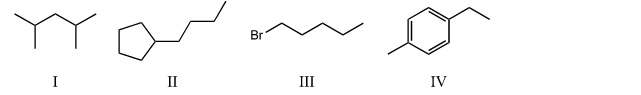Problem: Which compound below would give rise to 5 signals in the proton NMR spectrum and 7 signals in the carbon NMR spectrum? (Assume you can separate and see all peaks.) A) I B) II C) III D) IV E) None of the above.

FREE Expert Solution
97% (186 ratings)
Problem Details

Which compound below would give rise to 5 signals in the proton NMR spectrum and 7 signals in the carbon NMR spectrum? (Assume you can separate and see all peaks.)

A) I

B) II

C) III

D) IV

E) None of the above.What scientific concept do you need to know in order to solve this problem?

Our tutors have indicated that to solve this problem you will need to apply the 13C NMR: Cumulative Practice concept. If you need more 13C NMR: Cumulative Practice practice, you can also practice 13C NMR: Cumulative Practice practice problems .

What is the difficulty of this problem?

Our tutors rated the difficulty of Which compound below would give rise to 5 signals in the pro... as medium difficulty.

How long does this problem take to solve?

Our expert Organic tutor, Chris took 5 minutes to solve this problem. You can follow their steps in the video explanation above.

What professor is this problem relevant for?

Based on our data, we think this problem is relevant for Professor West's class at FAU.